1644391379

# Orthogonal Matrices and Subspaces: Orthonormal Columns in Q Give Q'Q = I

## Matrix Methods in Data Analysis, Signal Processing and Machine Learning

### Orthonormal Columns in Q Give Q'Q = I

This lecture focuses on orthogonal matrices and subspaces. Professor Strang reviews the four fundamental subspaces: column space C(A), row space C (A^T), nullspace N(A), left nullspace N (A^T).

#matrix #dataanalysis #machinelearning

## Buddha Community1644391379

## Matrix Methods in Data Analysis, Signal Processing and Machine Learning

### Orthonormal Columns in Q Give Q'Q = I

This lecture focuses on orthogonal matrices and subspaces. Professor Strang reviews the four fundamental subspaces: column space C(A), row space C (A^T), nullspace N(A), left nullspace N (A^T).

#matrix #dataanalysis #machinelearning1626057017

## What is GIVE Token (GIVE) | What is Givetoken (GIVE)

GIVEToken (GIVE) is a community-driven, fair-launched DeFi project built on HECO Chain #HECO. Main functions occur during each trade:

• Reflection
• LP Acquisition
• Burn.
• Charity

GiveToken brings the most revolutionary mechanism to the HECO ecosystem:

• Earn \$HT just by holding \$GIVE token
• GIVE \$HT for donate charity

Total Supply: 1 Quadrillion \$GIVE (1,000,000,000,000,000)

• 0.5% for creator & promotor
• 4.5% for foundation & developer
• 2% for marketing with referral program
• 1% for marketing with airdrop & holding NUT program
• 1% for operation & internal management
• 1% for listing fee (MDEX)
• 90% for the fair launch and locked

10% tax on transactions

• 4.5% of every transaction contributes toward automatically generating further liquidity
• 4.5% of every transaction is taken and re-distributed to all GIVE holders in \$HT
• 0.5% to the charity wallet, which donates on a weekly basis.
• 0.5% used as a marketing fund, through the referral program from NUT DEX

## Tokenomics

With a charity wallet set aside from the initial supply, and an aggressive transaction tax distribution, \$GIVE allows you to earn big as you hodlNUT DEX give 900 HT to create the first locked liquidity.

• NUT DEX use 900 HT to buy 90% GIVE total supply
• Create a first LP: GIVE - HT with 900HT & 900,000,000,000,000 GIVE. After that NUT team will locked this LP, by remove owner of this LP

Anti Pump-Dump-Exit Whales

• Transactions (sell/buy/transfer) that trade more than 0.01% of the total supply will be rejected.
• If whales want to make a transfer (between 2 wallets) that is larger than 0.01% of the total supply, they can use the Disruptive Transfer feature: the transfer will be charged for 2 HTLiquidity Locks

On 24 June, at the initial launch, we locked all LPs:

• 90% for the fair launch and locked
• NUT DEX use 900 HT to buy 90% GIVE total supply
• Create a first LP: GIVE - HT with 900HT & 900,000,000,000,000 GIVE. After that NUT team will locked this LP, by remove owner of this LP

#### Earn HT

You collect HT by accessing the dashboard page at https://givetoken.co

4.5% of every transaction is taken and re-distributed to all GIVEToken holders in \$HT.

You will have to wait for a specific duration called cycle (about 1 week) to collect your HT reward.

In this waiting duration, if you continue to add more than 2% (threshHoldTopUpRate) of your \$ balance, the waiting duration will proportionally add up with the cycle.

(If you sell \$GIVE it does not affect your cycle)

For example, on the first day of your cycle, you add up more 5% \$GIVE , you have to wait: *5%7days = 8,4 hours more. That means you can collect HT after 7 days and 8,4 hours.

But if the threshHoldTopUpRate >= 100%, the waiting duration will add up max to 7 days.

For example, on the first day of your cycle, you add up more 100% \$GIVE , you have to wait: 7 days more. That means you can collect HT after 14 days.

This will prevent cheaters who try to add more \$GIVE at the end of each cycle.

Collect HT Tax

If you collect more than 1 HT, 20% of it will be used to buy back \$GIVE at the market price and burn them immediately. This feature helps deflate the supply and increase the price of \$GIVE for long-term \$GIVE holders.

#### How and Where to Buy GIVE token?

GIVE has been listed on a number of crypto exchanges, unlike other main cryptocurrencies, it cannot be directly purchased with fiats money. However, You can still easily buy this coin by first buying Bitcoin, ETH, USDT, BNB from any large exchanges and then transfer to the exchange that offers to trade this coin, in this guide article we will walk you through in detail the steps to buy GIVE token.

You will have to first buy one of the major cryptocurrencies, usually either Bitcoin (BTC), Ethereum (ETH), Tether (USDT), Binance (BNB)…

We will use Binance Exchange here as it is one of the largest crypto exchanges that accept fiat deposits.

Binance is a popular cryptocurrency exchange which was started in China but then moved their headquarters to the crypto-friendly Island of Malta in the EU. Binance is popular for its crypto to crypto exchange services. Binance exploded onto the scene in the mania of 2017 and has since gone on to become the top crypto exchange in the world.

Once you finished the KYC process. You will be asked to add a payment method. Here you can either choose to provide a credit/debit card or use a bank transfer, and buy one of the major cryptocurrencies, usually either Bitcoin (BTC), Ethereum (ETH), Tether (USDT), Binance (BNB)…

Step by Step Guide : What is Binance | How to Create an account on Binance (Updated 2021)

Next step - Transfer your cryptos to an Altcoin Exchange

Since GIVE is an altcoin we need to transfer our coins to an exchange that GIVE can be traded. Below is a list of exchanges that offers to trade GIVE in various market pairs, head to their websites and register for an account.

Once finished you will then need to make a BTC/ETH/USDT/BNB deposit to the exchange from Binance depending on the available market pairs. After the deposit is confirmed you may then purchase GIVE from the exchange.

The top exchange for trading in GIVE token is currently Stellarterm and MDEX

🔺DISCLAIMER: The Information in the post isn’t financial advice, is intended FOR GENERAL INFORMATION PURPOSES ONLY. Trading Cryptocurrency is VERY risky. Make sure you understand these risks and that you are responsible for what you do with your money.

🔥 If you’re a beginner. I believe the article below will be useful to you ☞ What You Should Know Before Investing in Cryptocurrency - For Beginner

⭐ ⭐ ⭐The project is of interest to the community. Join to Get free ‘GEEK coin’ (GEEKCASH coin)!

☞ **-----https://geekcash.org-----**⭐ ⭐ ⭐

#blockchain #bitcoin #give #give token1666770774

## Variables in Python

In this Python tutorial for beginners, we learn about Variables in Python. Variables are containers for storing data values. A Python variable is a symbolic name that is a reference or pointer to an object.

## Creating Variables

Python has no command for declaring a variable.

A variable is created the moment you first assign a value to it.

### Example

``````x = 5
y = "John"
print(x)
print(y)``````

Variables do not need to be declared with any particular type, and can even change type after they have been set.

### Example

``````x = 4       # x is of type int
x = "Sally" # x is now of type str
print(x)``````

## Casting

If you want to specify the data type of a variable, this can be done with casting.

### Example

``````x = str(3)    # x will be '3'
y = int(3)    # y will be 3
z = float(3)  # z will be 3.0``````

## Get the Type

You can get the data type of a variable with the `type()` function.

### Example

``````x = 5
y = "John"
print(type(x))
print(type(y))``````

## Single or Double Quotes?

String variables can be declared either by using single or double quotes:

### Example

``````x = "John"
# is the same as
x = 'John'``````

## Case-Sensitive

Variable names are case-sensitive.

### Example

This will create two variables:

``````a = 4
A = "Sally"
#A will not overwrite a``````

## What is a Variable in Python?

A Python variable is a reserved memory location to store values. In other words, a variable in a python program gives data to the computer for processing.

### Python Variable Types

Every value in Python has a datatype. Different data types in Python are Numbers, List, Tuple, Strings, Dictionary, etc. Variables in Python can be declared by any name or even alphabets like a, aa, abc, etc.

In this tutorial, we will learn,

• How to Declare and use a Variable
• Re-declare a Variable
• Concatenate Variables
• Local & Global Variables
• Delete a variable

## How to Declare and use a Variable

Let see an example. We will define variable in Python and declare it as “a” and print it.

``````a=100
print (a)``````

## Re-declare a Variable

You can re-declare Python variables even after you have declared once.

Here we have Python declare variable initialized to f=0.

Later, we re-assign the variable f to value “guru99”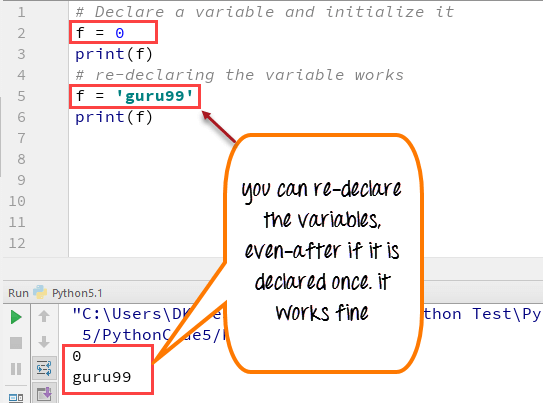Python 2 Example

``````# Declare a variable and initialize it
f = 0
print f
# re-declaring the variable works
f = 'guru99'
print f``````

Python 3 Example

``````# Declare a variable and initialize it
f = 0
print(f)
# re-declaring the variable works
f = 'guru99'
print(f)``````

## Python String Concatenation and Variable

Let’s see whether you can concatenate different data types like string and number together. For example, we will concatenate “Guru” with the number “99”.

Unlike Java, which concatenates number with string without declaring number as string, while declaring variables in Python requires declaring the number as string otherwise it will show a TypeError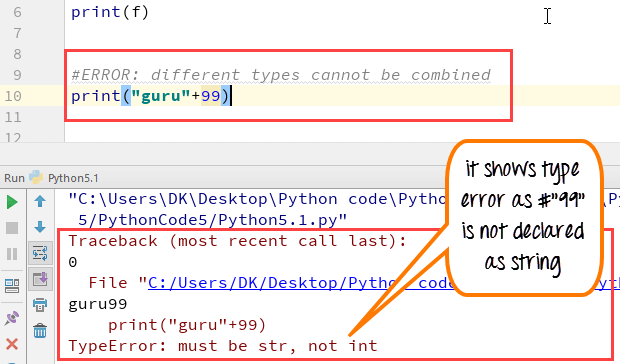For the following code, you will get undefined output –

``````a="Guru"
b = 99
print a+b``````

Once the integer is declared as string, it can concatenate both “Guru” + str(“99”)= “Guru99” in the output.

``````a="Guru"
b = 99
print(a+str(b))``````

## Python Variable Types: Local & Global

There are two types of variables in Python, Global variable and Local variable. When you want to use the same variable for rest of your program or module you declare it as a global variable, while if you want to use the variable in a specific function or method, you use a local variable while Python variable declaration.

Let’s understand this Python variable types with the difference between local and global variables in the below program.

1. Let us define variable in Python where the variable “f” is global in scope and is assigned value 101 which is printed in output
2. Variable f is again declared in function and assumes local scope. It is assigned value “I am learning Python.” which is printed out as an output. This Python declare variable is different from the global variable “f” defined earlier
3. Once the function call is over, the local variable f is destroyed. At line 12, when we again, print the value of “f” is it displays the value of global variable f=101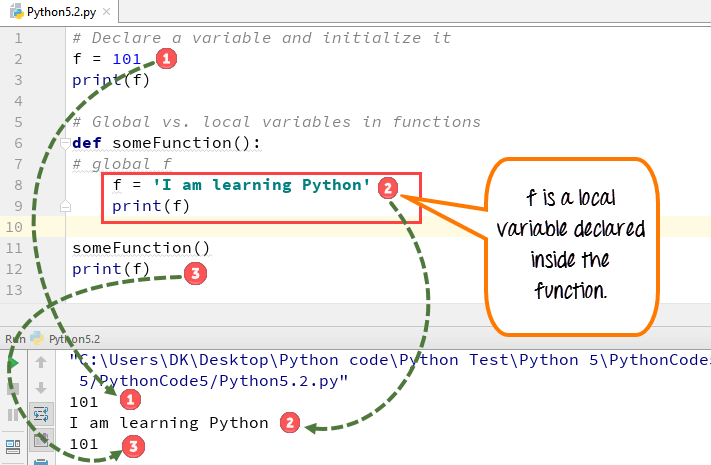Python 2 Example

``````# Declare a variable and initialize it
f = 101
print f
# Global vs. local variables in functions
def someFunction():
# global f
f = 'I am learning Python'
print f
someFunction()
print f``````

Python 3 Example

``````# Declare a variable and initialize it
f = 101
print(f)
# Global vs. local variables in functions
def someFunction():
# global f
f = 'I am learning Python'
print(f)
someFunction()
print(f)``````

While Python variable declaration using the keyword global, you can reference the global variable inside a function.

1. Variable “f” is global in scope and is assigned value 101 which is printed in output
2. Variable f is declared using the keyword global. This is NOT a local variable, but the same global variable declared earlier. Hence when we print its value, the output is 101

We changed the value of “f” inside the function. Once the function call is over, the changed value of the variable “f” persists. At line 12, when we again, print the value of “f” is it displays the value “changing global variable”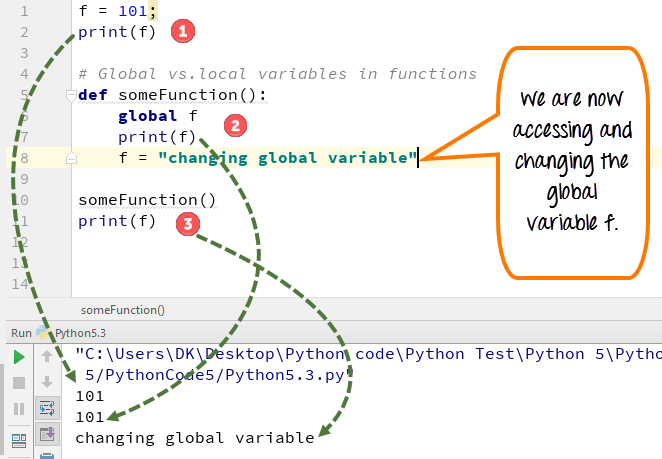Python 2 Example

``````f = 101;
print f
# Global vs.local variables in functions
def someFunction():
global f
print f
f = "changing global variable"
someFunction()
print f``````

Python 3 Example

``````f = 101;
print(f)
# Global vs.local variables in functions
def someFunction():
global f
print(f)
f = "changing global variable"
someFunction()
print(f)``````

## Delete a variable

You can also delete Python variables using the command del “variable name”.

In the below example of Python delete variable, we deleted variable f, and when we proceed to print it, we get error “variable name is not defined” which means you have deleted the variable.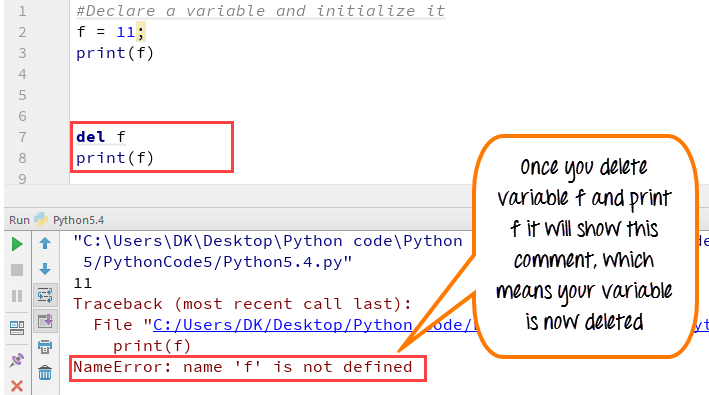Example of Python delete variable or Python clear variable :

``````f = 11;
print(f)
del f
print(f)``````

## Summary:

• Variables are referred to “envelop” or “buckets” where information can be maintained and referenced. Like any other programming language Python also uses a variable to store the information.
• Variables can be declared by any name or even alphabets like a, aa, abc, etc.
• Variables can be re-declared even after you have declared them for once
• Python constants can be understood as types of variables that hold the value which can not be changed. Usually Python constants are referenced from other files. Python define constant is declared in a new or separate file which contains functions, modules, etc.
• Types of variables in Python or Python variable types : Local & Global
• Declare local variable when you want to use it for current function
• Declare Global variable when you want to use the same variable for rest of the program

To delete a variable, it uses keyword “del”.

## A Beginner’s Guide To Python Variables

A variable is a fundamental concept in any programming language. It is a reserved memory location that stores and manipulates data. This tutorial on Python variables will help you learn more about what they are, the different data types of variables, the rules for naming variables in Python. You will also perform some basic operations on numbers and strings. We’ll use Jupyter Notebook to implement the Python codes.

Variables are entities of a program that holds a value. Here is an example of a variable:

x=100

In the below diagram, the box holds a value of 100 and is named as x. Therefore, the variable is x, and the data it holds is the value.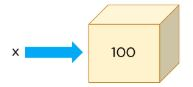The data type for a variable is the type of data it holds.

In the above example, x is holding 100, which is a number, and the data type of x is a number.

In Python, there are three types of numbers: Integer, Float, and Complex.

Integers are numbers without decimal points. Floats are numbers with decimal points. Complex numbers have real parts and imaginary parts.

Another data type that is very different from a number is called a string, which is a collection of characters.

Let’s see a variable with an integer data type:

``x=100``

To check the data type of x, use the type() function:

``type(x)``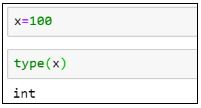Python allows you to assign variables while performing arithmetic operations.

``````x=654*6734
type(x)``````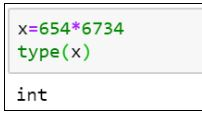To display the output of the variable, use the print() function.

``print(x) #It gives the product of the two numbers``

Now, let’s see an example of a floating-point number:

``````x=3.14
print(x)``````

type(x) #Here the type the variable is float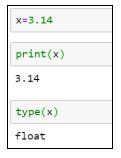Strings are declared within a single or double quote.

``````x=’Simplilearn’

print(x)

x=” Simplilearn.”

print(x)

type(x)``````

In all of the examples above, we only assigned a single value to the variables. Python has specific data types or objects that hold a collection of values, too. A Python List is one such example.

Here is an example of a list:

``````x=[14,67,9]

print(x)

type(x)``````

You can extract the values from the list using the index position method. In lists, the first element index position starts at zero, the second element at one, the third element at two, and so on.

To extract the first element from the list x:

``print(x)``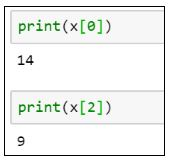To extract the third element from the list x:

``print(x)``

Lists are mutable objects, which means you can change the values in a list once they are declared.

``````x=70 #Reassigning the third element in the list to 70

print(x)``````

Earlier, the elements in the list had [14, 67, 9]. Now, they have [14, 67, 70].

Tuples are a type of Python object that holds a collection of value, which is ordered and immutable. Unlike a list that uses a square bracket, tuples use parentheses.

``````x=(4,8,6)

print(x)

type(x)``````

Similar to lists, tuples can also be extracted with the index position method.

``print(x) #Give the element present at index 1, i.e. 8``

If you want to change any value in a tuple, it will throw an error. Once you have stored the values in a variable for a tuple, it remains the same.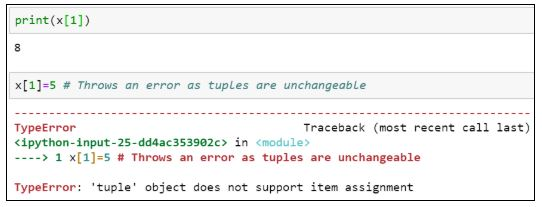When we deal with files, we need a variable that points to it, called file pointers. The advantage of having file pointers is that when you need to perform various operations on a file, instead of providing the file’s entire path location or name every time, you can assign it to a particular variable and use that instead.

Here is how you can assign a variable to a file:

``````x=open(‘C:/Users/Simplilearn/Downloads/JupyterNotebook.ipynb’,’r’)

type(x)``````

Suppose you want to assign values to multiple variables. Instead of having multiple lines of code for each variable, you can assign it in a single line of code.

``(x, y, z)=5, 10, 5``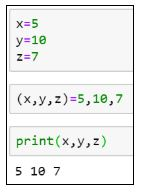The following line code results in an error because the number of values assigned doesn’t match with the number of variables declared.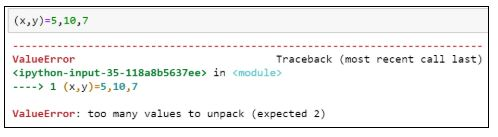If you want to assign the same value to multiple variables, use the following syntax:

``x=y=z=1``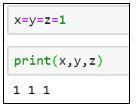Now, let's look at the various rules for naming a variable.

1. A variable name must begin with a letter of the alphabet or an underscore(_)

Example:

``````abc=100 #valid syntax

_abc=100 #valid syntax

3a=10 #invalid syntax

@abc=10 #invalid syntax``````

. The first character can be followed by letters, numbers or underscores.

Example:

``````a100=100 #valid

_a984_=100 #valid

a9967\$=100 #invalid

xyz-2=100 #invalid``````

Python variable names are case sensitive.

Example:

``````a100 is different from A100.

a100=100

A100=200``````

Reserved words cannot be used as variable names.

Example:

``````break, class, try, continue, while, if

break=10

class=5

try=100``````

Python is more effective and more comfortable to perform when you use arithmetic operations.

The following is an example of adding the values of two variables and storing them in a third variable:

``````x=20

y=10

result=x+y

print(result)``````

Similarly, we can perform subtraction as well.

``````result=x-y

print(result)``````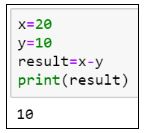Additionally, to perform multiplication and division, try the following lines of code:

``````result=x*y

print(result)

result=x/y

print(result)``````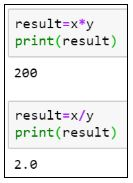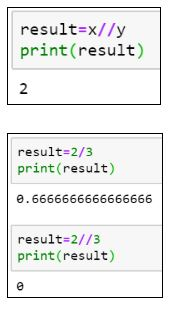As you can see, in the case of division, the result is not an integer, but a float value. To get the result of the division in integers, use “//”the integer division.

The division of two numbers gives you the quotient. To get the remainder, use the modulo (%) operator.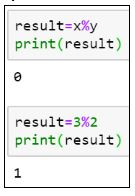Now that we know how to perform arithmetic operations on numbers let us look at some operations that can be performed on string variables.

``var = ‘Simplilearn’``

You can extract each character from the variable using the index position. Similar to lists and tuples, the first element position starts at index zero, the second element index at one, and so on.

``````print(var) #Gives the character at index 0, i.e. S

print(var) #Gives the character at index 4, i.e. l``````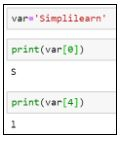If you want to extract a range of characters from the string variable, you can use a colon (:) and provide the range between the ones you want to receive values from. The last index is always excluded. Therefore, you should always provide one plus the number of characters you want to fetch.

``print(var[0:3]) #This will extract the first three characters from zero, first, and second index.``

The same operation can be performed by excluding the starting index.

``print(var[:3])``The following example prints the values from the fifth location until the end of the string.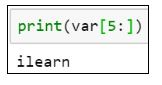Let’s see what happens when you try to print the following:

``print(var[0:20]) #Prints the entire string, although the string does not have 20 characters.``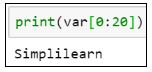To print the length of a string, use the len() function.

``len(var)``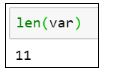Let’s see how you can extract characters from two strings and generate a new string.

``````var1 = “It’s Sunday”

var2 = “Have a great day”``````

The new string should say, “It’s a great Sunday” and be stored in var3.

``````var3 = var1[:5] + var2[5:13] + var1[5:]

print(var3)``````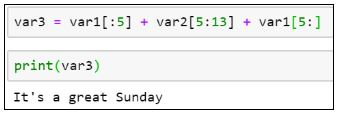Get prepared for your next career as a professional Python programmer with the Python Certification Training Course. Click to enroll now!

## Conclusion

I hope this blog helped you learn the concepts of Python variables. After reading this blog, you may have learned more about what a variable is, rules for declaring a variable, how to perform arithmetic operations on variables, and how to extract elements from numeric and string variables using the index position.

#python #programming1624431580

## How to add a new column to Pandas DataFrame?

In this tutorial, we are going to discuss different ways to add a new column to pandas data frame.

### What is a pandas data frame?

Pandas data frameis a two-dimensional heterogeneous data structure that stores the data in a tabular form with labeled indexes i.e. rows and columns.

Usually, data frames are used when we have to deal with a large dataset, then we can simply see the summary of that large dataset by loading it into a pandas data frame and see the summary of the data frame.

In the real-world scenario, a pandas data frame is created by loading the datasets from an existing CSV file, Excel file, etc.

But pandas data frame can be also created from the listdictionary, list of lists, list of dictionaries, dictionary of ndarray/lists, etc. Before we start discussing how to add a new column to an existing data frame we require a pandas data frame.

#pandas #dataframe #pandas dataframe #column #add a new column #how to add a new column to pandas dataframe1625889060

## Comprehensive Guide To Deep Q-Learning For Data Science Enthusiasts

This article will talk about reinforcement learning (RL) and Deep Q-Learning using openAI’s Gym environment and TensorFlow 2, and we will implement a case study using python. I assume that readers have a good understanding of reinforcement learning and deep learning. For the very beginners, I recommend this article before going further.

### Introduction to Reinforcement Learning

Reinforcement is a part of machine learning concerned about the action, which an agent in an environment takes to maximize the rewards. Reinforcement Learning differs from supervised learning and unsupervised learning in the sense that it does not need a supervised input/output pair. And not requires a higher amount of correction in any actions to make action highly efficient.

Register

Let’s say I want to make a bot(agent) playing ludo with the other three players with ludo dice(environment); this bot should have the ability to roll the dice (state) and picking up the right token(action), and moving the token based on dice number(rewards).

For a better understanding, you can learn reinforcement learning in depth from here.

In all reinforcement learning subjects, the **Markov Decision Process (MDP) **plays a huge role; an important point to notice is that each state presented in the environment results from its previous state, which is also a result of its previous state. Thus, somewhere, the present state of any environment results from the composition of information gathered from the previous states.

So the task of any agent is to perform an action and make a higher reward provided by the environment. The Markov Decision Process makes an agent decide to choose an optimal action on a given state to maximize the reward. The probability of choosing action at a particular time from a state is called policy. So the goal of the Markov Decision Process is to find the optimal policy.

### Introduction to Q-Learning Algorithm

In the figure, we can see that the processes of Q-Learning, from the start to the end of the processes, Q-Learning follow four methods and two sub-process. So, let’s discuss the details of every process.

1. Initialize parameter – In this step, the model learns about the action and states that an agent needs to perform in a certain environment and time.
2. Identify current state – An agent needs to store the previous records to act optimally to earn maximized rewards. To act in the current state, it needs to identify the state and perform a combination of actions.
3. **Choose an action and gain experience ** – By the initialisation process, a Q-table gets generated where it gives the information about the combination of actions and states. Then, it looks for past experiences and compares the weight. If it’s a new situation, the Q-Table will update it for the next step.
4. **Update the reward in Q-table and determine the next state – **After gaining the experience, agents get the reward from the environment. That reward amplitude gets recorded in the Q-table as experience data, and this becomes helpful in predicting the actions in the next step.

#developers corner #deep q-learning #q-learning #q-learning #data science